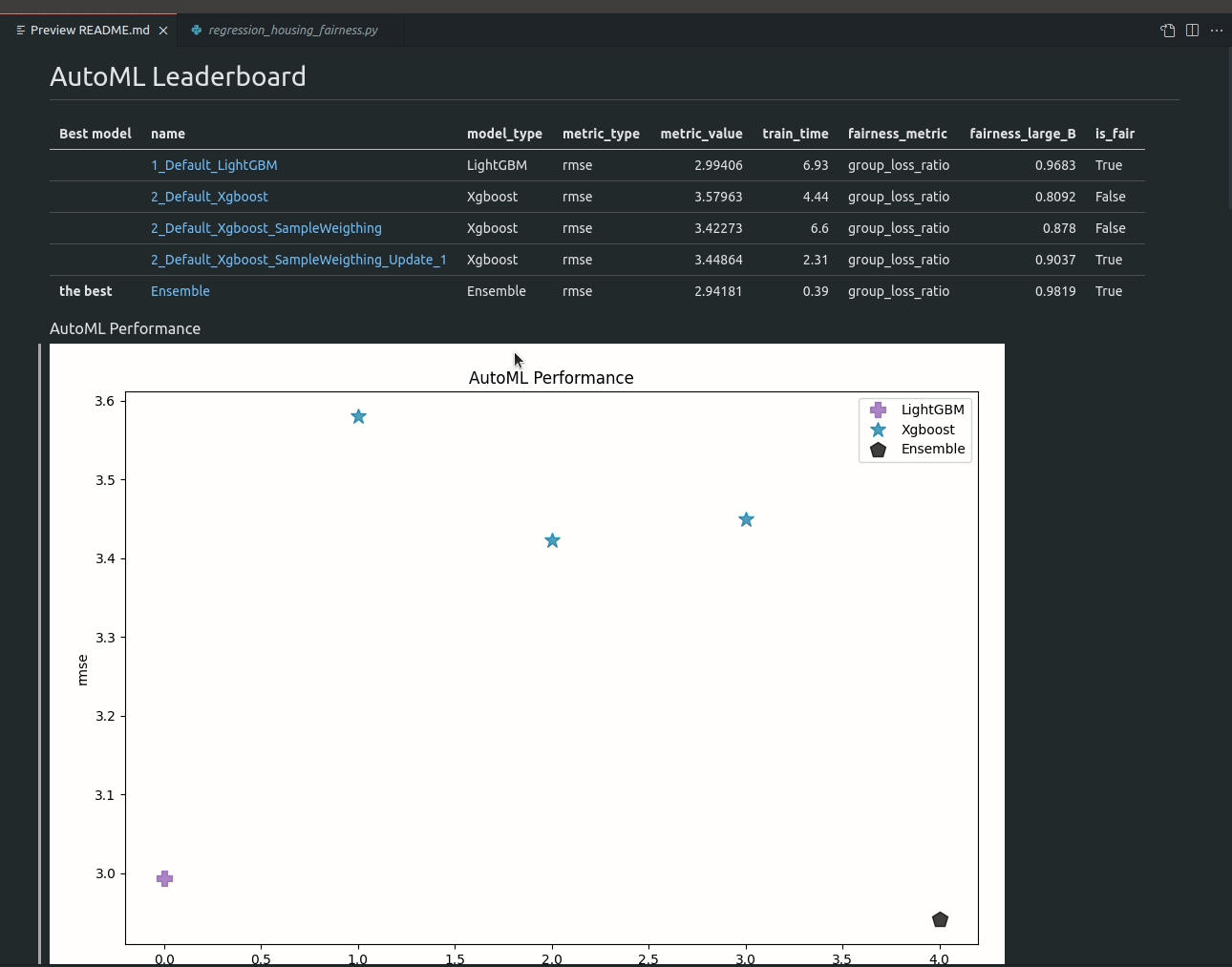# Fairness aware AutoML on regression task¶

Please run the example script to train AutoML on Housing dataset with `large_B` as sensitive feature.

``````python examples/scripts/regression_housing_fairness.py
``````

Code from example script:

``````import numpy as np
import pandas as pd
from supervised.automl import AutoML

x_cols = [c for c in df.columns if c != "MEDV"]

df["large_B"] = (df["B"] > 380) * 1
df["large_B"] = df["large_B"].astype(str)

print(df["large_B"].dtype.name)
sensitive_features = df["large_B"]

X = df[x_cols]
y = df["MEDV"]

automl = AutoML(
algorithms=["Xgboost", "LightGBM"],
train_ensemble=True,
fairness_threshold=0.9,
)
automl.fit(X, y, sensitive_features=sensitive_features)

df["predictions"] = automl.predict(X)
``````

The example report with fairness metrics reported for each model generated automatically by AutoML: